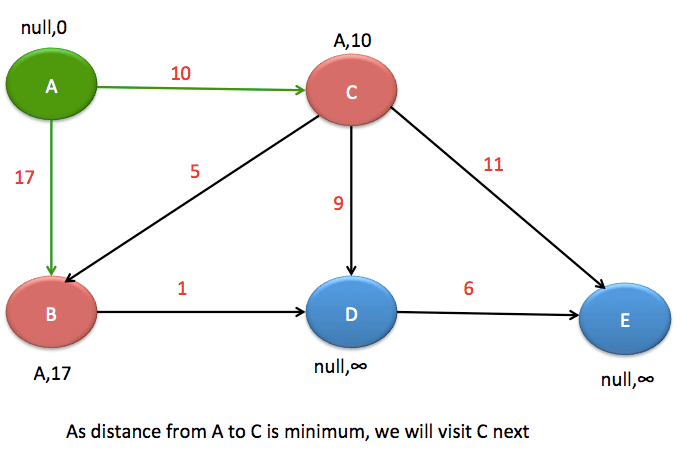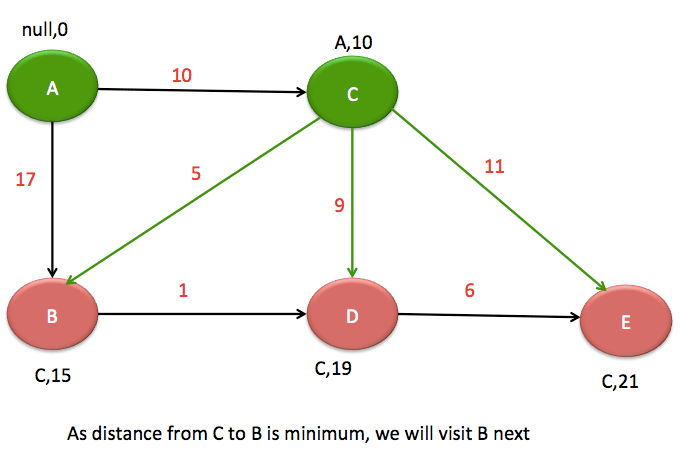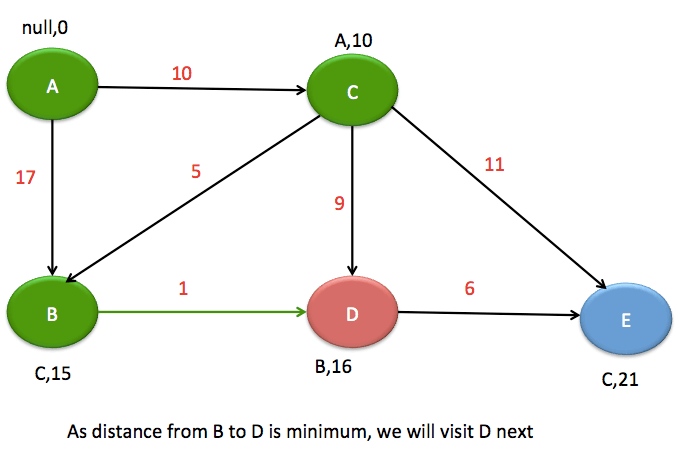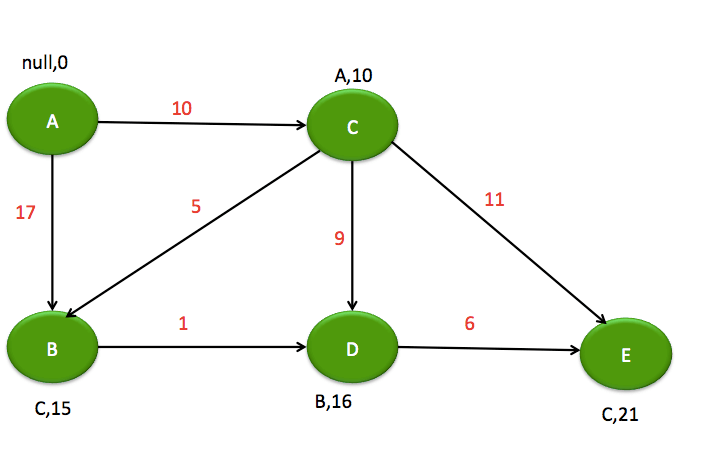# Dijkstra’s algorithm in java

If you want to practice data structure and algorithm programs, you can go through Java coding interview questions.

In this post, we will see Dijkstra algorithm for find shortest path from source to all other vertices.

## Problem

You will be given graph with weight for each edge,source vertex and you need to find minimum distance from source vertex to rest of the vertices.

## Algorithm

There will be two core classes, we are going to use for Dijkstra algorithm.
Vertex: This class contains name, visited flag, predecessor(To track the short path, so that we can backtrack) and distance from source node and also the list of outgoing edge from this vertex.
Edge: This class contains Source vertex, target vertex, and weight.

Let’s first understand how are we going to solve it visually.As you can see, we have initialized predecessor and minimum distance to default.Green node represents visited nodes and red color represent neighbors of the vertex.Here we are considering :

Total distance from source vertex A to vertex B via vertex C: 15

Total distance from source vertex A to vertex D via vertex C: 19

Total distance from source vertex A to vertex E via vertex C: 21

As distance from C to B is minimum i.e 15, that’s why we have chosen vertex B as next vertex.As you can see, we are done with Dijkstra algorithm and got minimum distances from Source Vertex A to rest of the vertices.

Let me go through core algorithm for Dijkstra.

• Set distance for source Vertex to 0.
• Set distance for all other vertices to infinity.
• At each vertex, we need to choose a node with minimum distance from source vertex and we are going to use priority queue for that.
• Add source node to PriorityQueue.
• Do the following when PriorityQueue is not empty
• Poll vertex (Let’ say vertex A) from the PriorityQueue.
• Iterate over neighbours(Let’s say Vertex B) for the vertex A.
• Calculate new distance for vertex B by adding vertex.getDistance and edge.getWeight
• If new distance <  Vertex B’s current distance then update the node(Distance,predecessor) in PriorityQueue.

Let’s see complete source code for Dijkstra’s algorithm

## Dijkstra’s algorithm example

Create a class named Vertex.java as below:

Create another class named Edge.java as below.

Create another class named "DijkstraShortestPath.java" as below. This will contain main Dijkstra algorithm.

Create another class named "DijkstraMain.java". This class will contain main function to run the algorithm.

When you run above program, you will get below output:

======================================
Calculating minimum distance
======================================
Minimum distance from A to B: 15.0
Minimum distance from A to C: 10.0
Minimum distance from A to D: 16.0
Minimum distance from A to E: 21.0
===================== =================
Calculating Paths
======================================
Shortest Path from A to B: [A, C, B] Shortest Path from A to C: [A, C] Shortest Path from A to D: [A, C, B, D] Shortest Path from A to E: [A, C, E]

That’s all about Dijkstra algorithm in java.

## Related Posts

•26 September

### Print all subarrays of a given array

Table of ContentsProblemSolution If you want to practice data structure and algorithm programs, you can go through 100+ data structure and algorithm programs. In this post, we will see how to print all subarrays of given array. Problem Print all print all subarrays of given array. For example: If array is {1,2,3} then you need […]

•16 September

### Doubly Linked List in java

If you want to practice data structure and algorithm programs, you can go through Java coding interview questions. In this post, we will see about Doubly LinkedList implementation in java. We have already seen the implementation of singly linked list. You can consider this as an extension of Singly linked list.It is quite complex to implement […]

•22 October

### Trie data structure in java

If you want to practice data structure and algorithm programs, you can go through 100+ java coding interview questions. In this post, we will see about trie data structure in java. What is Trie : Trie is data structure which stores data in such a way that it can be retrieved faster and improve the performance. […]

•12 October

### Implement Queue using Linked List in java

If you want to practice data structure and algorithm programs, you can go through 100+ java coding interview questions. In this post , we will see how to implement Queue using Linked List in java. Queue is abstract data type which demonstrates First in first out (FIFO) behaviour. We will implement same behaviour using Array. Although java provides implementation […]

•10 October

### Convert sorted array to balanced binary search tree

Table of ContentsAlgorithm:Java code to convert sorted array to balanced binary search tree: If you want to practice data structure and algorithm programs, you can go through 100+ java coding interview questions. In this post ,  we will see how to convert sorted array to balanced binary search tree. Algorithm: You might know that inorder traversal of […]

•23 September

### Sort a Stack using another stack

Table of ContentsProblemSolution :Java codeComplete Java program to sort a stack using addition stack: If you want to practice data structure and algorithm programs, you can go through 100+ java coding interview questions. In this post,  we will see how to sort a stack using another stack. Problem Given a Stack,  you need to sort it […]

1.Victor Basic says: